Frequency Selective Distortion in Case of Mimo Transmission

Dr. Vijay Tiwari*

Citation: Frequency Selective Distortion in Case of Mimo Transmission. American Research Journal of Computer Science and Information Technology, Vol 2, no. 1, 2017; pp:1-9.

Copyright This is an open access article distributed under the Creative Commons Attribution License, which permits unrestricted use, distribution, and reproduction in any medium, provided the original work is properly cited.

Abstract:

Use of OFDM over multiple carriers is in use for some time now. Multiple carriers’ performance relies on the diversity. In case of diversity with multiple receive and transmit antennas, every antenna receives a linear combination of individual transmissions and added noise. However concept of channel decoupling by using Single Value Decomposition (SVD) has been employed to ensure decoupling among MIMO channels. In this paper we have shown that a decoupled channel behaves as if undergoing spatial multiplexing. Transmission without distortion is always a priority and hence concept of coherence bandwidth has been considered and its effects on the channel bandwidth are reported. Doppler Shift effects over MIMO channel are discussed. Dependence of BER on SNR has been simulated for various cases where in combinations of multiple transmission and reception antennas have been discussed.

Keywords: SIMO, MISO, SNR, Coherence bandwidth, Single Value Decomposition, MIMO

Description:

INTRODUCTION

For the sake of estimation, let me consider power requirement in wired and wireless channels to achieve BER of 10-6. To achieve BER of 10-6 in any wireless channel SNR of 57 dB is needed whereas for the same BER wired channel needs only 13.6 dB. Hence wireless channel needs whooping 10000 times more power than the wired counterpart to deliver same performance. Such a critical disadvantage was attributable to the phenomenon of deep fade i.e. a condition when signal power is less than the noise power at the receiver. Hence it is the probability of deep fade that determines the performance of wireless systems. Probability of Deep fade event = 1/ (SNR). Multiple Input and Multiple Output (MIMO) employ multiple streams to achieve higher data rates with reasonably low fading probability, almost negligible. Multiple transmit and receive antennas add up the mush desired SNR after considering a decoupled channel. This paper proposes a time-division multipleaccess. An adaptive modulation scheme with dynamic channel assignment (AMDCA) was proposed in . This paper aimed at achieving higher capacity systems under the constraints of dynamic shift in propagation path varying traffic conditions. It was based on C/(N+ I) ratio for every TDMA slot with a view to reach an optimum modulation by use of spatially distributed parameters. As an augmented approach references have been made in  which proposes to tweak the cell shapes in form of microcells as cross-shaped cells and cigar-shaped cells. It has made an attempt to include user mobility with an overall aim to increase the capacity. Bolotin  reported that channel throughput drops significantly for an exponential distribution of call holding time. Then the effort further moved on to consider challenge of channel assignment and in multi-channel multi-radio Wireless Mesh Networks (WMNs) as reported in .

We may consider input to MIMO channel a ‘t’ dimensional transmit vector and ‘r’ dimensional receive vector . In fact the MIMO channel will transform from ‘t’ dimensional to ‘r’ dimensional vector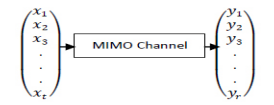Hence MIMO channel could be represented in the form of ‘r x t’ matrix. Each element of the matrix is referred as flat fading channel coefficient. is channel coefficient between ith receive antenna and jth transmit antenna and there will be a total of r x t channel coefficients.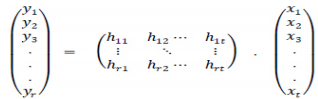Streamlining the coverage increases the coverage area and hence number of users go up. Beam forming is employed in the IEEE standard 802.11n  and other communication standards like IEEE 802.16. Improvements to IEEE 802.11 (introduction of beam forming) successfully achieved higher data rates . 802.16 define fixed broadband standards popularly known as Stanford University Interim (SUI) channel models. Detailed working of IEEE 802.16 is described in . Capacity of the OFDM based spatial multiplexed systems was analysed in .

SISO (Single Input Single Output)

Diversity order of ZF is given as r + t -1. For single receive antenna (r = 1) and single transmit antenna (t =1), the system reduces to a Rayleigh Flat Fading case.

MIMO (Multiple Input Multiple Output)

MIMO system can be modeled as y = H. x +n; Noise is assumed to be isotropic (spatially white noise) i.e. equally distributed in all directions. H is a channel matrix. Considering case where ‘Number of receive antennas r > Number of transmit antennas t’ and if we assume inversion of channel matrix at the receiver as: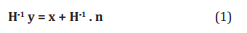We may end up in a situation where the inverse of channel matrix does not exist. There will thus be a need to arrive at a more generalized expression. If x̅ is the average transmit vector, we need to calculate minimum error vector by minimizing norm of error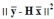known as least squares solution. Here y̅ is measured at the receive antenna and H x̅ is known after suitable modeling of the channel. The above expression can be split to :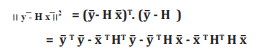Differentiating the above equation with respect to ‘x’ and equating it equal to zero will reveal minima; we get the estimated value of transmitted symbol for minimum error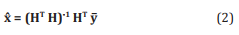x̂ is the estimate of transmitted symbol ‘x’ at the receiver given the channel ‘H’ and also a condition that number of receive antennas are greater than number of transmit antennas. This is an approximate solution that minimizes the least squares error. This is known as Zero Forcing receiver (ZF).

MMSE receivers have minimum least square error and more robust against the channel noise. We may use Hamiltonian operator over the equation (2) and employ concept of a pseudo-inverse of H, which exits if the inverse of H exists and reduces to identity matrix of dimension ‘t’ (‘t’ is the number or transmit antennas). We may take a separate approach where transmitted symbols are taken as random variable and mean error is also modeled in the form of a random variable. The problem of estimating error reduces to estimating the scalar quantity ‘x’ given ‘r’ vector measurements (of received symbols ‘y’) at different receive antennas. For this purpose a linear estimator is employed to combine the vector measurements. Estimation x̂ = CT y̅, where CT is a linear combiner and y̅ are various measurements. Aim is to select linear combiner such that squared error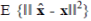is minimum. Hence linear estimator should minimize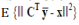. Expected squared error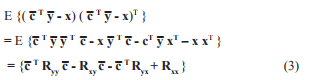Here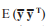is covariance of matrix y = [y1 , y2 …..yr ]. Similarly,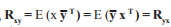are cross covariance. So equation (3) will reduce to an expression for a combiner as under:-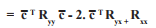For a minimum error in combiner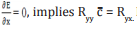. Hence optimum value of c̅ to minimize mean square error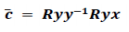indicating value of linear minimum mean squared error associated with the transmitted symbol ‘x’: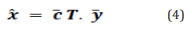which is applicable to channel in matrix notation. For complex notation, LMMSE receiver can be represented as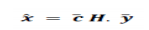in another case of vectors the LMMSE receiver can be represented as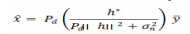Even as h tends to zero MIMO MMSE estimator does not enhance noise. is known as regularization term and bounds the noise. Hence LMMSE is superior to ZF receivers. At high SNR LMMSE turns to be ZF receiver and at low SNR turns to be matched filter.

Coherence Bandwidth

Multiple transmitter and receiver antennas alongwith various reflected paths add to severe crisis of delays in arrival of transmitted symbols. Hence delays of the order of μS are a reality and should be taken care of t=by the MIMO receiver.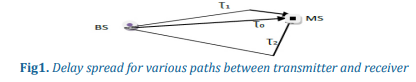Delay spread in frequency domain is also known as Coherence bandwidth. If we have a channel function H(f) in frequency domain then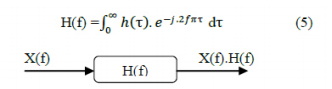Considering the time delay in time domain which can be transformed in to frequency domain as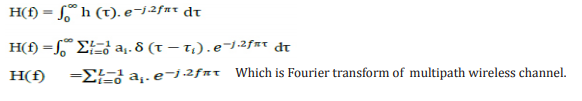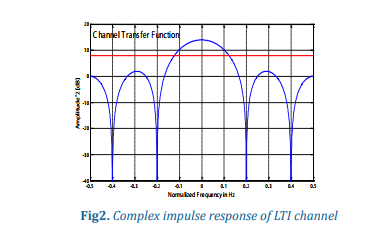A channel could be modeled as a Linear Time Invariant system where received symbol is convolution of transmitted symbol and channels response. Change in the position of antenna results in Phase change. Impulse response of the channel is shown in Fig.2.

Let coherence bandwidth of a channel be given as Bc and Signal bandwidth as Bs. In general Bs <= Bc, (spectrum of signal is limited to coherence bandwidth) for output without distortion. Hence it behaves as if a flat fading channel.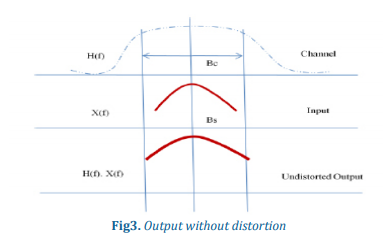Frequency selective distortion

In case when Bs > Bc then distortion occurs. It is termed as frequency selective distortion.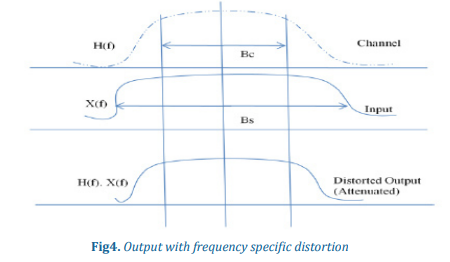Inverse Discrete Fourier Transform (IDFT) converts the symbol in to a wave form that is used as OFDM sub-channel (Fig. 5) whose frequency is an integer number of cycles.

DECOUPLING OF CHANNEL

As transmit symbols are available to all the receive antennas, estimating the value of receive symbol becomes critical and cumbersome. Single Value Decomposition (SVD) is one of the techniques employed to estimate transmitted symbol at the receiver.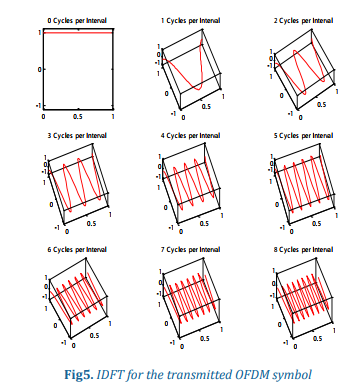In case we replace the channel characteristics in MIMO channel matrix, and encode at the transmitter: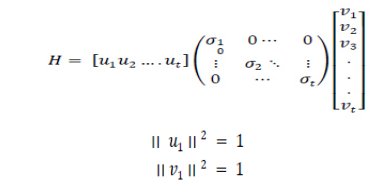Here are known as singular values and ‘t’ is number of transmit antennas. And also i.e. they are arranged in ordered. Also number of non-zero singular values is equal to the rank of the matrix. To get receive beam forming vector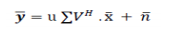Multiply both side by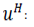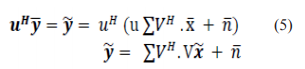At the receiver the vector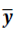is multiplied with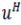and we simplify the expression as: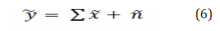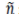is a noise vector and transmit vector is represented as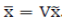. So at the receiver we multiply the vector with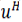and at the receiver we pre-code the vector

Hence after the SVD along with precoding system can be represented as: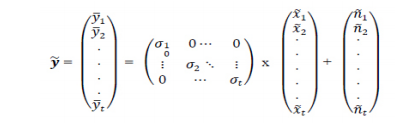So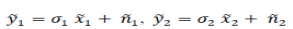and so on. As every transmitted symbol interferes at each receive antenna. However in the transformed space, the transmit symbol is available only at i th receive antenna,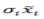appears only once. Hence channels are effectively decoupled. This may be referred as parallelization of MIMO system. As ‘t’ transmit symbols are being transmitted in parallel and hence it may also be referred as spatial multiplexing.

Temporal Spatio White Noise

In case of MIMO, noise is considered as r dimensional vector as each of the ‘r’ receive antennas receive different amount of noise which are uncorrelated across antennas and time. Assuming power of noise component at each receive antenna is . Since the noise samples are uncorrelated and these are Gaussian hence: . Covariance matrix of noise in the system is expressed as follows: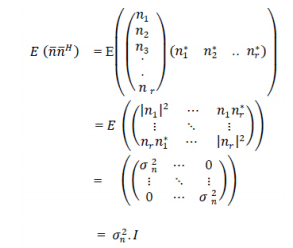that is why the noise is termed as spatially white. It is equally distributed in all directions. It is isotropic in nature. Since the noise is uncorrelated across all the receive antennas hence it is termed as spatially uncorrelated.

SNR Dependence

Channel capacity is directly dependent on the SNR. It can be computed as log2 (1 + SNR). MIMO channel can accommodate up to: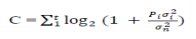In order to increase the channel capacity C, the total power at transmitter should be divided in such a way that inequality Pi≤P  is satisfied for ith channel.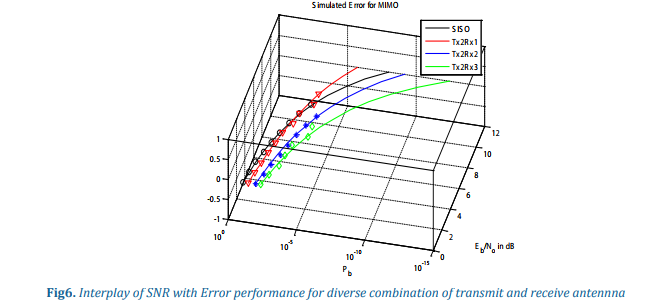Interse variation among the received SNR as compared with the simulated error performance coupled with number of transmit and receive antennas is shown in Fig. 7. The improvement in the error performance with increase in diversity is evident.

Doppler Shift

Channel experience variations and some of these can be attributed to Doppler shift. Frequency variation as described in , depends on the relative velocity of receiver/Transmitter (v), and inclination of line of sight from direction of motion (θ):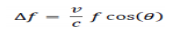Path loss can be described as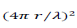where r is the distance and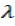is wavelength.

Equation 2.3 does not take into account relativistic properties such as time dilation and so the equality is an approximation. The received signal is superposition of various sinusoids. When Sinusoids add constructively the graph in Fig.7, grows.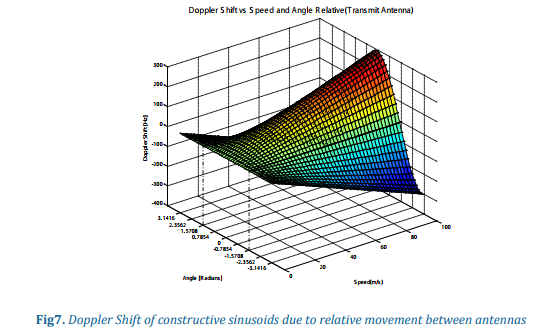CONCLUSION

MIMO transmission is affected by the diversity and SNR dependence on BER and diversity was established. Doppler shift has a slow varying impact over the SNR. OFDM are represented as an IDFT wave forms which represented as sub channels.

References

1. Takehiro Ikeda, Seiichi Sampei and Norihiko Morinaga, “TDMA-Based Adaptive Modulation with Dynamic Channel Assignment for High-Capacity Communication Systems”, IEEE Transactions on Vehicular Technology, Vol. 49, No.

2, March 2000. 2. Ho-Shin Cho, Min Young Chung, Sang Hyuk Kang, and Dan Keun Sung, “Performance Analysis of Cross- and Cigar-Shaped Urban Microcells Considering User Mobility Characteristics” IEEE Transactions on Vehicular Technology, Vol. 49, No. 1, January 2000.

3. Yuguang Fang and Imrich Chlamtac, “Teletraffic Analysis and Mobility Modeling of PCS Networks” IEEE Transactions on Communications, Vol. 47, No. 7, JULY 1999.

4. Andrew Brzezinski, Gil Zussman and Eytan Modiano, “Distributed Throughput Maximization in Wireless Mesh Networks via Pre-Partitioning” IEEE/ACM Transactions on Networking, Vol. 16, No. 6, December 2008.

5. Enhancements for higher throughput, IEEE Std 802.11n-2009 (Amendment to IEEE Std 802.11-2007 as amended by IEEE Std 802.11k-2008, IEEE Std 802.11r-2008, IEEE Std 802.11y-2008, and IEEE Std 802.11w- 2009), (2009), pp. c1 –502.

6. Perahia, Cordeiro, Park and Yang, IEEE 802.11ad: Defining the Next Generation Multi-Gbps Wi-Fi, in Consumer Communications and Networking Conference, Jan. 2010, IEEE, pp. 1-5.

7. V. Erceg, K.V.S Hari and M. S. Smith, “Channel Models for Fixed Wireless Applications,” IEEE 802.16 Broadband Wireless Access Working Group Report - 03/01, June 2003.

8. H. Bolcskei, G. Gesbert, and A. J. Paulraj “On the Capacity of OFDM-based Spatial Multiplexing Systems,” IEEE Transactions on Communications, Vol. 50, No. 2, pp. 225-234, February 2004.

9. S Yang, L Wang, T Lv, L Hanzo, Approximate Bayesian probabilistic-data association-aided iterative detection for MIMO systems using arbitrary M-ary modulation. IEEE Trans. Veh. Tech. 62(3), 1228–1240 (2013).

10. D. TSE AND P. VISWANATH, Fundamentals of Wireless Communication, Cambridge University Press, Cambridge, UK, 2005.

TestimonialsChicago

• 8770 West Bryn Mawr Ave, Suite 1300
Chicago, Illinois - 60631-3515, USA.
• +1-773-649-9077
• +1-773-649-5196

Arkansas

• 1120 S Walton Blvd, Suite 138
Bentonville, Arkansas - 72712-0077, USA.
• +1-479-696-9120
• +1-479-696-9132